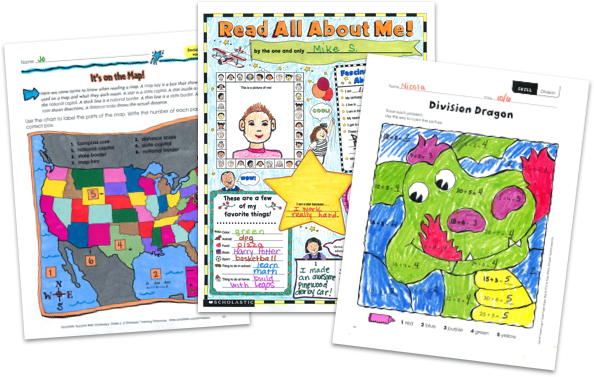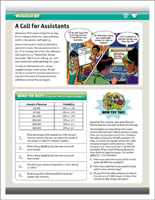MY FILE CABINETScholastic Teachables (formerly Scholastic Printables) offers more than 200 seventh grade math worksheets that span more than 20 different math skills and more than 30,000 award-winning printables for all grades and subjects. Sign up today and get instant online access to 7th grade math worksheets, plus lesson plans, practice pages, and more to cover all the necessary math skills. Learn why thousands of teachers have chosen Scholastic to be their teaching resource.

Scholastic Teachables (formerly Scholastic Printables) offers more than 200 seventh grade math worksheets that span more than 20 different math skills and more than 30,000 award-winning printables for all grades and subjects. Sign up today and get instant online access to 7th grade math worksheets, plus lesson plans, practice pages, and more to cover all the necessary math skills. Learn why thousands of teachers have chosen Scholastic to be their teaching resource.

## Scholastic's 7th Grade Math Worksheets Cover:

• Algebra
• Averages
• Charts and Graphs
• Data Analysis
• Decimals
• Fractions
• Functions
• Geometry
• Inequalities
• Logic and Problem Solving
• Measures of Central Tendency
• Money
• Multiplication and Division
• Patterns
• Percent, Proportions, Rates, and Ratios
• Permutations
• Probability and Statistics
• Properties
• Ratio, Proportion, and Scale
• Real-World Math
• Simulations
• Unit Rates
• Word Problems
• And More!

## Sample 7th Grade Math Worksheets

Scholastic Teachables’ hundreds of printable 7th grade math worksheets are targeted for all your teaching needs. Plus, you’ll get access to more than 30,000 award-winning printables and leveled learning collections in reading and math to reach every learner. Scholastic publishes new worksheets each month to keep your courses fresh, exciting, and up-to-date. Spend more time focusing on your courses and less time on preparing materials—simply find it, print it, teach it!

Here's a sample of seventh grade activities and worksheets for you to try in your class—FREE with a 30-day trial or subscription.

## Scholastic's 7th Grade Math Worksheets Cover:

• Algebra
• Averages
• Charts and Graphs
• Data Analysis
• Decimals
• Fractions
• Functions
• Geometry
• Inequalities
• Logic and Problem Solving
• Measures of Central Tendency
• Money
• Multiplication and Division
• Patterns
• Percent, Proportions, Rates, and Ratios
• Permutations
• Probability and Statistics
• Properties
• Ratio, Proportion, and Scale
• Real-World Math
• Simulations
• Unit Rates
• Word Problems
• And More!
• Algebra
• Averages
• Charts and Graphs
• Data Analysis
• Decimals
• Fractions
• Functions
• Geometry
• Inequalities
• Logic and Problem Solving
• Measures of Central Tendency
• Money
• Multiplication and Division
• Patterns
• Percent, Proportions, Rates, and Ratios
• Permutations
• Probability and Statistics
• Properties
• Ratio, Proportion, and Scale
• Real-World Math
• Simulations
• Unit Rates
• Word Problems
• And More!

## Sample 7th Grade Math Worksheets

Scholastic Teachables’ hundreds of printable 7th grade math worksheets are targeted for all your teaching needs. Plus, you’ll get access to more than 30,000 award-winning printables and leveled learning collections in reading and math to reach every learner. Scholastic publishes new worksheets each month to keep your courses fresh, exciting, and up-to-date. Spend more time focusing on your courses and less time on preparing materials—simply find it, print it, teach it!

Here's a sample of seventh grade activities and worksheets for you to try in your class—FREE with a 30-day trial or subscription.## Additional Alphabet Worksheets (Subscription Required)

Try FREE for 30 days!

If you are not 100% satisfied cancel during the FREE trial and owe nothing. If you are not 100% satisfied cancel during the FREE trial and owe nothing.